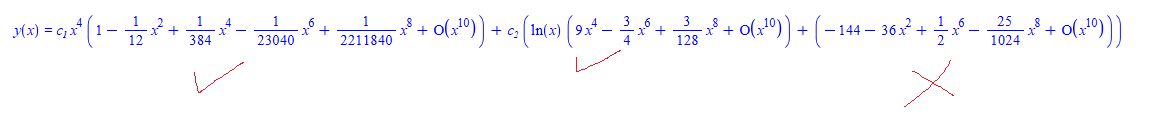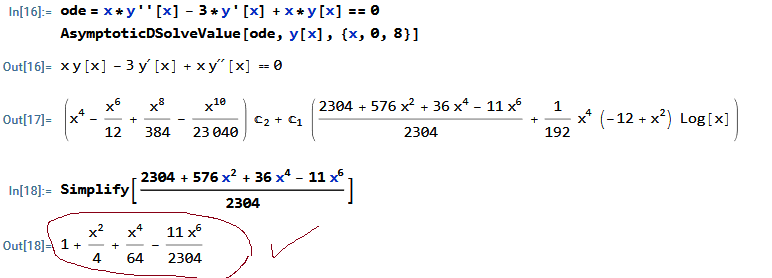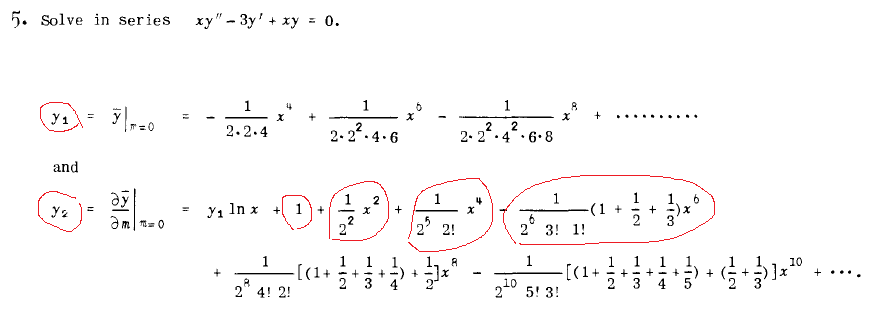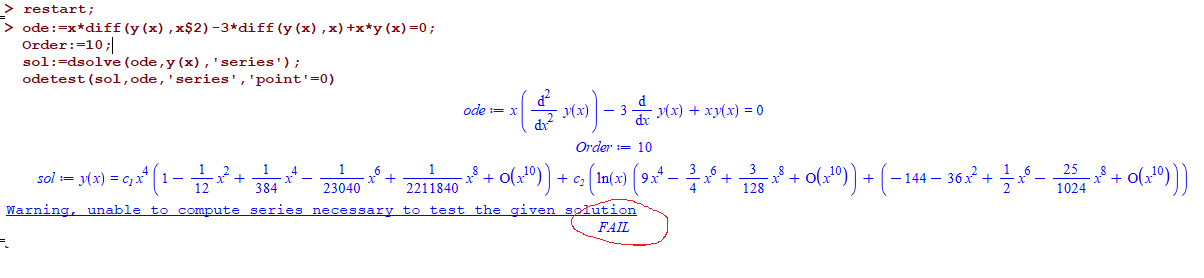# Question:possible wrong series solution for second order ode

## Question:possible wrong series solution for second order ode

Maple 2022

This is second order ode solved using series method. This problem from textbook. The solution given by Maple does not match the book. I also solved this by hand and my hand solution agrees with the text book. I also solved this using Mathematica and its solution agrees with the book.

Maple solution does not agree with the book for y2.  i.e. the general solution for this problem has the form

y= c_1 * y1 + c_2* (  y1 * ln(x)  +  y2 )

This is a Frobenius series method, since regular singular point and it falls into the hard case, where roots of indicial equation has difference of integer and where the second solution y2 can't be obtained using same method as y1 due to being undefined if using same method. So it require adjustment to the Frobenius series method.

This is ode

```restart;
Order:=10;
ode:=x*diff(y(x),x\$2)-3*diff(y(x),x)+x*y(x)=0;
dsolve(ode,y(x),'series')

```Maple says that

y2 = -144 - 36*x^2 + 1/2*x^6 - 25/1024*x^8 + ...)

First, it is missing x^4. And not able to make other coefficients match book. Book says y2 should be

y2 = 1 + x^2/4 + x^4/64 - (11 x^6)/2304 + ....

And that is what I get and also Mathematica:I do not have screen shot now of the page from the book to show. It is from an old textbook. Will try to make screen shot if needed.  This is problem 5, page 212 from SCHAUM's "differential equations" by Frank Ayers. 1952 edition. There is free PDF files on the net. Here is screen shotMy question is, why is Maple's series soluiton for the second basis solution y2 different? Could someone verify this? It also failes to verify it

```restart;
ode:=x*diff(y(x),x\$2)-3*diff(y(x),x)+x*y(x)=0;
Order:=10;
sol:=dsolve(ode,y(x),'series');
odetest(sol,ode,'series','point'=0)
```Update

I've testsed few more problems, solved by hand and verified using Mathematica. All these problems give wrong solution by Maple for y_2. At least the solutions do not match the book and do not match my hand solution and do not match Mathematica. In all cases Mathematica's solution and my hand solution match the book.

All these problem fall into the same difficult case of Frobenius series, where roots of indicial equation differ by integer and where y_2 can not be obtained directly using similar method used to obtain y_1. Other cases of Frobenius roots, Maple give complete correct general solutions. It is only this case where there seems to be something wrong.

In all of these problems below, y_1 solution is correct. It is the last series in y_2 shown which does not agree with book and it is this part which require using modifed method to obtain as explained on the book where these problems are solved from the above links. All the books used can be found online.

 > interface(version);> #problem  problem 5, page 212 from SCHAUM's "differential equations" by Frank Ayers. 1952 edition. ode:=x*diff(y(x),x\$2)-3*diff(y(x),x)+x*y(x)=0: sol:=dsolve(ode,y(x),'series'); odetest(sol,ode,'series','point'=0)Warning, unable to compute series necessary to test the given solution> #page 19, example 5. NASA report TR R-390. By Gabriel Allen (PDF online) ode:=x^2*diff(y(x),x\$2)+x*diff(y(x),x)+(x^2-4)*y(x)=0: sol:=dsolve(ode,y(x),'series'); odetest(sol,ode,'series','point'=0)Warning, unable to compute series necessary to test the given solution> # problem 20.6, page 114 from SCHAUM's "differential equations" by Richard Bronson. 1978 edition. ode:=x^2*diff(y(x),x\$2)+(x^2-2*x)*diff(y(x),x)+2*y(x)=0: sol:=dsolve(ode,y(x),'series'); odetest(sol,ode,'series','point'=0)Warning, unable to compute series necessary to test the given solution> # problem 20.7, page 115 from SCHAUM's "differential equations" by Richard Bronson. 1978 edition. ode:=x^2*diff(y(x),x\$2)+x*diff(y(x),x)+(x^2-1)*y(x)=0: sol:=dsolve(ode,y(x),'series'); odetest(sol,ode,'series','point'=0)Warning, unable to compute series necessary to test the given solution>